# Random NumbersWritten by

### Every so often some randomness is required in a design. But how can you generate randomness in a deterministic system like an electronic board or in software running on a microcontroller? Robert explains how.

• How can you generate randomness in a deterministic system like a board or in software running on an MCU

• What is “random”?

• How to plants a see in a random-generation algorithm

• How to use cryptographic algorithms

• How to use feedback registers

• How to use analog signals to produce randomness

• Proteus VSM from Labcenter Electronics

• Dieharder program

Welcome back to the Darker Side. Usually, the goal of a hardware or software designer is to build a solid-rock product with well-defined outputs whatever are the inputs. However, this is quite far from a common real-world experience. As Sidney Poitier said, “So much of life, it seems to me, is determined by pure randomness.” So, from time to time, some randomness—or at least pseudo-randomness—is required in a design. Electronic games come to mind, but the applications are numerous. Let’s consider an example.

In telecommunications, message collision avoidance protocols are often based on listen-before-talk algorithms. Typically, a node checks if the transmission channel is free before transmitting. If not, it waits for the channel to be ready, waits for a random time, checks again if the channel is still free, and, if so, transmits (see Figure 1). Such an algorithm drastically reduces the number of collisions on the channel when several nodes want to transmit at the same time, thanks to some randomness.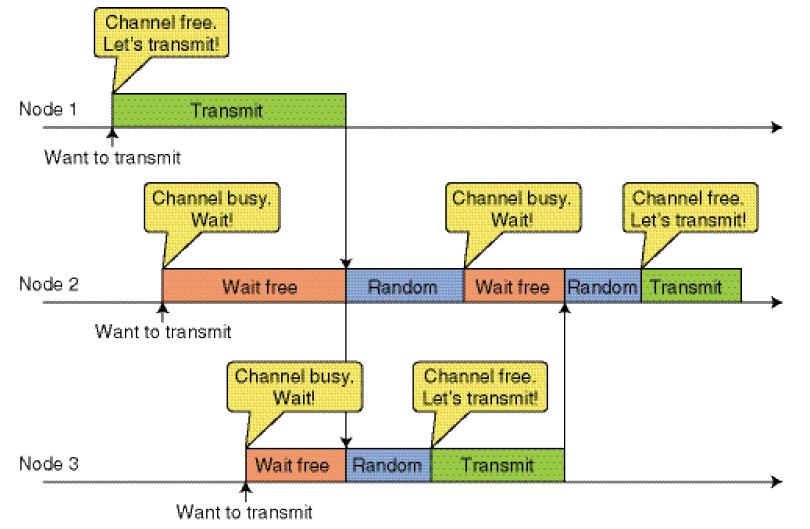Figure 1 An example of a listen-before-talk protocol, requiring some randomness

But how to generate randomness in a purely deterministic system like an electronic board or software running on a microcontroller or computer? If you think this is simple, keep reading as this is my monthly topic.

##### WHAT IS “RANDOM”?

Let’s assume you need to generate a suite of random integer numbers, from 0 to N–1. It could be a suite of decimal digits like 4-2-3-4-0-9-8 (with N = 10), a suite of binary digits like 1-1-0-0-1-0-1-1 (N = 2), or a series of 16-bit integers like 1223-17-65532-33442 (N = 216 = 65535) or whatever else.

What is a suite of random numbers? The question may seem obvious, but, well, it is not. Do you want a proof? Donald Knuth’s four-volume series, The Art of Computer Programming, is the best resource I know on the subject. The subject “random numbers” fills 200 pages of the second volume titled “Seminumerical Algorithms”—of which no less than 150 pages are devoted to the question of randomness. Of course, I won’t go into the same level of detail here. This is just an introduction.

Randomness can be evaluated by several tests, and a given suite of numbers can be accepted as random if, statistically, it satisfies all of them. Obviously, the first test is that each number must appear with the same probability. Statisticians have developed plenty of methods to verify that such occurrence statistics are not biased, like the well-know “Chi-square” test. But, clearly, this is not enough. 01010101 is not random, even if 1s and 0s have exactly the same occurrence ratio. We also want to avoid repetitions of any sequence of numbers, like in the previous example or in more complex sequences like 10-17-773-10-17-9253 or 1001101110110100110. This is a bit more difficult to specify. Is it enough? Of course not. We also need to avoid in fact any regular pattern, like in 1-4-7-10-13 (each number is the previous one plus three) or in 10-17-15-22-19-26-22-29. (Did you find it?) It’s not that easy.

##### THE WRONG METHODS

Defining a sequence of random numbers is complex, and developing a method to actually generate one is even harder than it seems. Donald Knuth himself confesses in his book that he was wrong while developing a super-complex random number generation algorithm. He programmed it, ran it, and immediately found that the algorithm always generated the same number after seconds!

If you have already developed your own random number generation routine, I strongly encourage you to download Dieharder, a program developed by Robert G. Brown at Duke University. (Refer to the Resources section of this article.) Dieharder is a random number generator testing suite. Feed it with your random numbers, and it will tell you if they are actually random. You may be surprised by the result.

In a nutshell, inventing your own method is probably a very bad idea. Even if you think you are clever, and you probably are, you may not be cleverer than the experts that have already developed some algorithms. Once again, as Knuth states, “Random numbers should not be generated with a method chosen at random.“

##### LET’S PLANT A SEED

As presented in the introduction, any digital design is (or should be) deterministic. This means that any software-based random number generator is a pseudorandom generator. If you run the same program twice with the same starting conditions, you will surely get the same list of “random” numbers. To counteract this problem, all random-generation algorithms use a seed, which must be defined by the programmer. A given value for the seed provides a given series of random numbers. For example, look at the libc standard C-library. Two functions are available: rand() and srand(). The former generates a new pseudorandom number each time it is called. The latter initializes the seed.

As illustrated in Figure 2, the seed defines the initial value of a variable which represents the current state of the algorithm. Let’s call it S and assume this is a 32-bit integer. This state S is then updated each time a new number is required, using a given function (f), and the random value is calculated from S using another function (g). So the generic pseudocode is the following:

``````S := Seed
For each new random number
S:=f(S)
Random:=g(S)
``````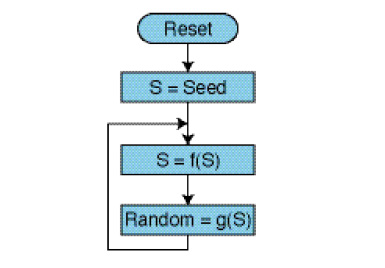Figure 2 A typical random number generation algorithm starts with a given seed and updates an internal state variable each time a new random value is required.

I will explain f and g. But wait, how does the seed initialize? To avoid repeating the same random numbers, this seed must be different each time the program is started. So we are back to the initial problem. We need a random seed! However, we only need it once, so constraints are a little relaxed. The only important thing is that it must be different each time the program is started. One of the common solutions is to use a human-related value (e.g., the number of microseconds between program start and a button press). If you can’t, you can use a unique value associated with each device, like its serial number, but then store the state of the random generator in nonvolatile memory and never reset it after the first run. It is not easy. But there are solutions.

##### ARITHMETIC SUITES

Which algorithms can be used to implement—that is, to actually generate pseudorandom values—the aforementioned f and g functions? As is often the case, very simple methods are usually the best. The ubiquitous algorithm to generate a list of pseudorandom integer numbers is called Linear Congruent Generator (LGC). It’s complex wording for a very simple method (see Figure 3). If you take the current value of S, multiply it by a fixed number (the multiplier A), add a fixed number (the increment C), and truncate the result using a modulo-M operation, you get the new value of S:

``S := f(S) = (A x S + C) mod M``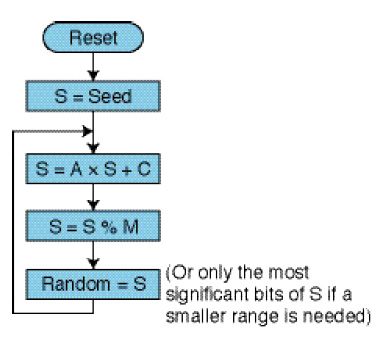Figure 3 A linear congruent generator simply uses arithmetic operations. You can easily implement it in any microcontroller or FPGA design using minimal resources.

Based on S, you can simply get your random number by either using S directly as a 32-bit random number or by truncating it if you need fewer bits. A note of caution: It might not be obvious, but the most significant bits (MSB) of S are more random than the low bits, so you’d better use the MSBs.

All of the abbreviations might be confusing, so refer to Listing 1 for an actual extract from the GCC Glibc rand() routine. As you can see, this code is exactly using the method I’ve presented, with quite exotic values for A and C. The modulo operation is done by a logical AND operation with 231, giving random numbers within the same range.

``````Listing 1
Code from the GCC Glibc rand() routine

int32_t val = state;
val = ((state * 1103515245) + 12345) & 0x7fffffff;
state = val;
*result = val;``````

You’re now wondering how to select adequate values for A, C, and M. Well, once again, this is not that simple. A “good” set of values will firstly insure that all possible 232 values of S are used before rolling back on the same numbers. Our friends the mathematicians proved this is the case if the following three conditions are satisfied. (That’s the Hull-Dobell Theorem. You can download the demonstration from the link provided in the Resources section of this article.) Condition 1: M and C are relatively prime. Condition 2: A-1 is divisible by all prime factors of M. Condition 3: A-1 is divisible by 4 if M is divisible by 4. However, these constraints only guarantee that the state S will go through all M possible values before rolling back, not that the output number would be effectively random enough. Fortunately, great thinkers have already worked the subject, and there are tables of “good” values for A, C, and M. Of course, the one used by LIBC are not bad, but other values are as good. Knuth’s book includes a table providing several good other candidates. If you are resource-constrained, you can even use a simpler method by using only a multiplication, meaning C = 0. For example the best option for 32-bit numbers found by L. C. Killingbeck seems to be the following:

``S := (S x 2650845021) mod 2^32``

A modulo 232 operation is basically to throw away the MSBs, so this operation can easily be implemented by a 32 × 32 bit multiplication and it will run in a single cycle on most 32-bit microcontrollers.

##### CRYPTOGRAPHY TO THE RESCUE

Such linear congruent methods have the advantage of simplicity. However, there are better methods if you are ready to pay the price in terms of the number of instructions and complexity. How? Using cryptographic techniques, for instance. By nature, all cryptographic algorithms are very good random generators, simply because a ciphered text is supposed to look random. So if you take any data which is never the same (it could be a simple counter) and pass it through a ciphering algorithm, you will get something random. It would even be better if you run the ciphering algorithm again and again on the same data block. This is exactly what the well-known AES ciphering algorithm does using a variant called AES-OFB (Output FeedBack). This mode, as illustrated on Figure 4, is a very strong pseudorandom generator and could be very easy to implement in microcontrollers including a hardware-assisted AES engine.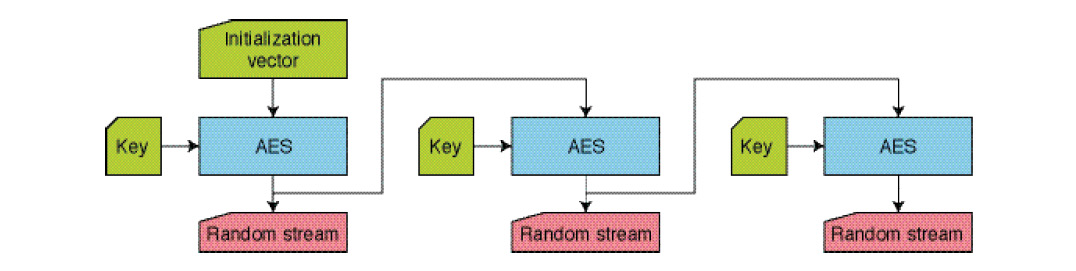Figure 4 AES ciphering can be used to generate a random stream through the Output Feedback (OFB) mode.
##### FEEDBACK REGISTERS

Want another nice random generation method? This one is dedicated to bit stream generation—that is, the generation of a random sequences of 1s and 0s. The concept of Linear-Feedback Shift Register (LFSR) is very straightforward, and you will understand that it is closely related to the arithmetic methods presented above.

An LFSR is a shift register those input is a logic combination of its outputs. This combination is usually made by taking some of the shift register’s outputs, called “taps,” and an exclusive-OR (XOR) gate. At each clock cycle, the content of the register is shifted right by 1 bit, and the left-most bit takes a new value from the XOR gate (see Figure 5).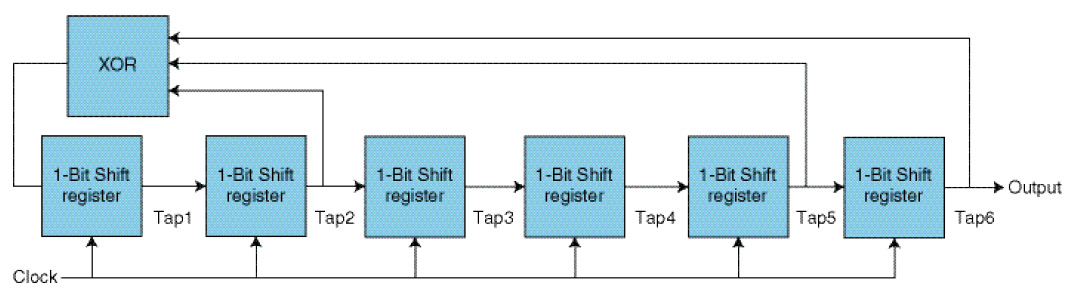Figure 5 A Linear Feedback Shift Register (LFSR) is a binary shift register with some feedback gates. With proper selection of the taps positions, the output bits are pseudorandom.

Of course, as before, the initial value of the shift register defines in a deterministic way the se the -1 is important because an LFSR always has one disallowed state” quence of 1s and 0s, so it must be initialized with a different seed value if different sequences are required. Nothing new here.

What about the taps? A shift register with a length of n bits can only have 2N different states, so it will surely roll over and generate the same bit stream after a while. However, “good” choices for the taps will ensure that the rollover will not be sooner. The output of the LFSR will have a period of 2N – 1 clock pulses. (The -1 is important because an LFSR always has one disallowed state.) Once again, you might not be surprised to know that some engineers have already worked it out for you. You will easily find tables giving adequate taps for LFSR’s ranging from 2 to 168 bits in length. One is included in Peter Alfke’s application note, “Efficient Shift Registers, LFSR Counters, and Long Pseudo-Random Sequence Generators” (Xilinx, 1996).

Just for fun, I used the tap values from the table to design an 8-bit LFSR using some 74-series logic gates. I drafted the schematics with Labcenter’s Proteus program (which includes the VSM mixed-mode simulator), hit Run, and got the waveform illustrated in Figure 6. Seems random, right?Figure 6An 8-bit LFSR simulated under Proteus VSM(click to enlarge)
##### ANALOG TO THE RESCUE

Last but not least, a good source of randomness is the analog world. This is, in fact, usually more of a nuisance than a feature, but here the randomness of nature can help. If your product has an actual sensor, you could use it as a random source. Do you have a light sensor, vibration sensor, noise sensor, or something similar? Convert its output to a numeric value using a high-resolution analog-to-digital converter, take the LSBs, and they will probably be nicely random. If I remember correctly, Circuit Cellar columnist Jeff Bachiochi once used this method with a Geiger cell, as radioactive disintegrations are random by nature.

The same analog random method can be used online. If your product is connected to the Internet, you might find an online analog-based random number generator! Take a look at www.random.org. These guys provide true random numbers to anyone, using atmospheric radiofrequency noise as a source of randomness.

Just a caution here. An analog source may not be as random as you think. Firstly, even if it is random, your ADC might not be that perfect. It is, for example, common to have ADCs that have different probability of occurrence of LSBs, especially those using successive approximation techniques. Moreover, the randomness of an analog source is much more Gaussian-shaped than uniform, simply because its noise is the sum of several actual noise sources, and the sum of a large number of random variables is always a Gaussian. (I guess I would need another column to dig into that subject.) So, as usual, you should use this method with care.

##### WRAPPING UP

Here we are. I’ve only scratched the surface of this subject. That’s why you should have a look at the Resources section of this article. Browse to the listed websites or read The Art of Computer Programming. And even more importantly, go practice! That’s the best way to bring random generation techniques into the light.RESOURCES
P. Alfke, “Efficient Shift Registers, LFSR Counters, and Long Pseudo-Random Sequence Generators,” XAPP052, Xilinx, 1996, www.xilinx.com/support/documentation/application_notes/xapp052.pdf.
R. Brown, “Dieharder: A Random Number Test Suite,” Ver. 3.31.1, 2016, www.phy.duke.edu/~rgb/General/dieharder.php.
T. Hull and A. Dobell, “Random Number Generators,” SIAM Review, Vol. 4, No. 3, 1962, http://chagall.med.cornell.edu/BioinfoCourse/PDFs/Lecture4/random_number_generator.pdf
D. Knuth, The Art of Computer Programming, Third Edition, Addison-Wesley, 2011.

SOURCE
Proteus VSM
Labcenter Electronics | www.labcenter.com

PUBLISHED IN CIRCUIT CELLAR MAGAZINE • JUNE 2016 #311 – Get a PDF of the issue

 Keep up-to-date with our FREE Weekly Newsletter! Don't miss out on upcoming issues of Circuit Cellar. Subscribe to Circuit Cellar Magazine Note: We’ve made the May 2020 issue of Circuit Cellar available as a free sample issue. In it, you’ll find a rich variety of the kinds of articles and information that exemplify a typical issue of the current magazine. Would you like to write for Circuit Cellar? We are always accepting articles/posts from the technical community. Get in touch with us and let's discuss your ideas.